# Newton’s Second Law of Motion

• Difficulty Level : Hard
• Last Updated : 16 Jun, 2021

The phenomenon which governs the change of position of a body over a specified time frame is known as motion. Motion is mathematically evaluated in terms of the position vectors, that is, displacement, distance, and speed factors, that is, velocity, acceleration, speed, and time. Through the naked eye, the motion of a body can be visualized by determining the change in the positional coordinates of the body with respect to time and attaching this frame of reference to an arbitrary observer.

Branch of physics concerned with movement or motion of bodies with no reference to its cause is popularly known as kinematics. The branch dealing with the forces and their corresponding effect noticed on the motion of objects is known as dynamics

### Newton’s Laws of Motion

The branch of physics dealing with the simulated motions of all large-scale and familiar bodies in the universe, for instance, the cars, planets, and humans come under classical mechanics, stipulated by Newton. In comparison, the motion of minutely small atomic and sub-atomic objects is covered under the branch of quantum mechanics, stipulated by Euler.

The following are Newton’s Laws of Motion:

• Law of Inertia or Newton’s first law states that, if a body is at rest or moving at a constant speed in a straight line, it will remain at rest or keep moving in a straight line at constant speed unless it is acted upon by an external acting force.
• The second law states that the rate of change of momentum of a body is directly proportional to the force applied for any object of constant mass.
• The third law states that when one object applies a force on a second object, the second object exerts a force back on the first object that is equal in magnitude and opposite in direction.

### Newton’s Second Law of Motion

Isaac Newton’s second law of motion gives the relationship between the force and acceleration of any object in the universe. This postulate states that:

The rate of change of momentum of an object is proportional to the applied unbalanced force, in the direction of the force.

Mathematically, it is defined as:

Force ∝ Change in Momentum / Change in the Time Taken

or

F ∝ dp / dt

where F is the Force, dp is the change in momentum and dt is the change in the time taken.

Let’s study more about momentum before deriving the expression for second law of motion as:

### Momentum

Momentum is the quantity of motion, which is multiplied by the amount of matter moved and the velocity at which it moves. When you walk, run, etc., you have momentum. If a bicycle and a car both drive down the street at the same speed, the automobile will have more momentum. Because velocity is considered a vector, momentum is also considered a vector.

The momentum of a body is the product of the mass of the body and its associated velocity. Momentum can be considered to be a vector quantity, that is, it has both an associated magnitude and direction.

Mathematically, the momentum (p) of an object of mass (m) moving with velocity (v) is defined as:

p= m × v

The unit of momentum is kg ms-1.

The second law of motion gives us a method to measure the force acting on an object as a product of its mass and the associated acceleration.

### Derivation of Second law of Motion

The rate of change in momentum of a body is directly proportional to the applied force and occurs in the force’s direction. Newton’s first law gives a realistic representation of force, whereas Newton’s second law gives a quantitative description of force.

Let us now consider a body with instantaneous velocity, and momentumwhich is given by,Since, according to the second law of motion,where \vec{F} is the force acting on the object.

Also, since the momentum is defined as,Therefore, the previous equation becomes,orwhere ‘k’ is the constant of proportionality.

As the mass m of a body can be considered to be a constant quantity so derivative is applicable to the velocity of the body as shown below,It is known that the time rate change of velocity of the body is termed as its acceleration i.e.Therefore,The units of force are also chosen such that ‘k’ equals one.

As a result, if a unit force is selected to be the force causing a unit acceleration in a unit mass, i.e.,

F = 1 N, m = 1 kg and a = 1 ms-2. This implies, k = 1.

Thus, the Newton’s second law of motion in mathematical form is given as,That is, the applied force of a body is defined as the product of its mass and acceleration. Hence, this provides us a measure of the force.

If F = 0, we get a = 0. This is similar to Newton’s first law of motion. That is, if there is no net external force, there will be no change in state of motion, implying that its acceleration is zero.For Changing Mass:

Let us assume a body to be at an initial point (0) specified at location L0 and at the time instance t0. Let us assume the body having mass m0 and traveling with a uniform velocity v0. On subjecting the body to a force F, the body moves to point 1, situated at the location L1 and reaches this point at time instance t1

The mass and velocity of the body undergoes transformation as the body travels to v1. Deriving the values for m1 and v1, we get,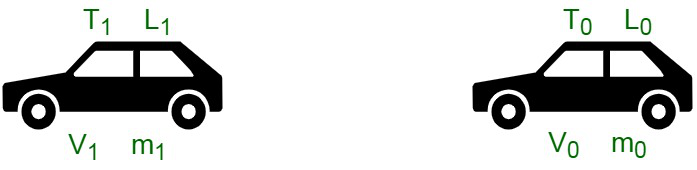For Constant Mass:

For a constant mass, the usage of Newton’s second law can be equated as follows:### Practical Applications of Newton’s Second Law of Motion

The second law of motion finds its usage in several domains like:

• On the cricket ground, the fielder pulls his hands in the backward direction to catch the fast approaching cricket ball. This reduces the momentum of the ball and induces a delay. When the ball comes in the hand of fielder and comes to a halt, the momentum of ball is reduced to zero. In case, the ball stops suddenly the momentum reaches 0 in an instant time frame. There is a quick rate of change in momentum due to which the player’s hand may get injured. Therefore, pulling the hand backward a fielder induces delay to the change of momentum to become zero, to prevent injury.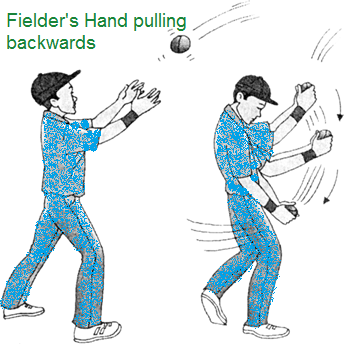• A sand bed or cushioned bed is provided to athletes performing long jumps to induce a delay in the change of momentum. The momentum induced because of the velocity and mass of the athlete is reduced to zero as soon as the athlete reaches back on the surface. In case the rate of momentum changes instantly, it may hurt the player. The purpose of cushioned bed is to delay the momentum of the athlete to zero and thus prevent injury.
• In case of sudden halt to the vehicle, that is stoppage due to braking or accidents, the passenger tends to be pushed in the direction of motion of vehicle and may get fatal injuries. In such a case, the change of momentum is reduced to zero. The stretchable seat belts increase the delay in the rate of momentum to be reduced to zero, to prevent injury.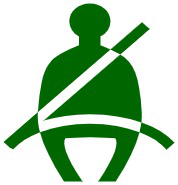• On kicking a ball, the person moves in the direction of the ball. In addition, the stronger the ball is kicked, the stronger the force we put on it and the further away it will travel.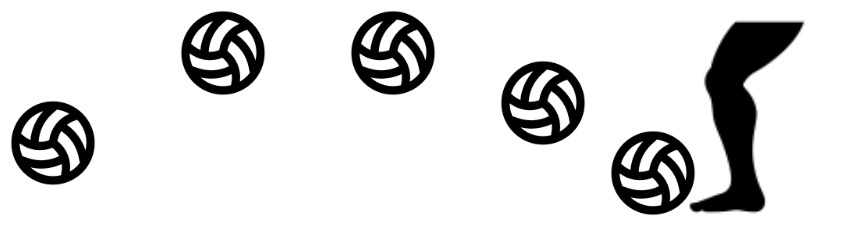• Acceleration of the rocket is due to the force applied to move the rocket, termed as thrust.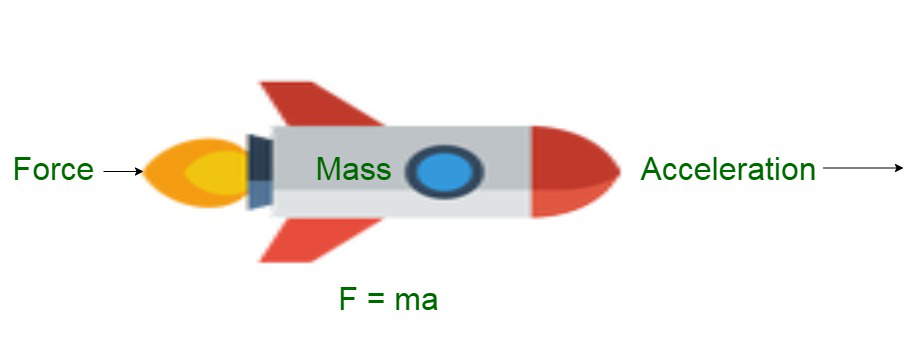• An object falling down from a certain height, undergoes an increase in acceleration because of the gravitational force applied.

### Sample Problems

Problem 1: If a bullet of mass 40 gm is shot from an Assault Riffle that has an initial velocity of 80 m/s the mass of the Assault Riffle is 15 kg. What is the initial recoil velocity of the Assault Riffle?

Solution:

Given that,

The mass of bullet (m1) = 40 gm or 0.04 kg.

The mass of the Assault Riffle (m2) = 15 kg.

The initial velocity (v1) = 80 m/s.

Therefore, according to the law of conservation of momentum,

0 = 0.04 × 80 + 15 × v

15 × v = -3.2

v = -3.2 / 15 m/s

= -0.21 m/s

Problem 2: If an object of mass 20 kg is moving with a constant velocity of 8 m/s on a friction-less ground. What will be the force required to keep the body moving with the same velocity?

Solution:

Given that,

The mass of the object (m) = 20 kg.

The acceleration of the object (a) = 0 (as object is moving constantly).

The applied force is given as,

F = m × a

= 20 kg × 0

= 0 N

Problem 3: If a heavy truck of weighing 2000 kg is running with some velocity. If the drive applies brakes is brought to rest, after application of brakes the heavy truck goes about 20 m when the average resistance being offered to it is 4000 N. What will be the velocity of the heavy truck engine?

Solution:

Given that,

The mass of the heavy truck (m) = 2000 kg.

The resistance or Force (F) offered by the ground = – 4000 N (as negative stopping force is applied)

The distance traveled after applying brakes (s) = 20 m.

The Final velocity (v) = 0 m/s (as the heavy truck was brought to rest)

The formula to calculate the applied force is,

F = m × a

where a is the acceleration.

Rearrange the above formula for a.

F = m × a

a = F / m

= – 4000 N / 2000 kg

= -2 m/s2

Now, use the equation of motion

v2 = u2 + 2as

0 = u2 + {2 × (-2) × 20}

u = 80 m/s

Example 4: A mini truck of 2500 kg with a velocity of v runs into with head on with a big truck of 5000 kg with a velocity of −v. Which truck will experience the greater force? Which experiences the greater acceleration?

Solution:

The mini truck and the big truck experience equal and opposite forces. But since the mini truck has a smaller mass it will experience greater acceleration than the big truck.

Therefore, according to the Newton’s second law of motion,

F = ma

or

a = F / m

Hence, the truck with greater mass’s acceleration will be decreased.

Problem 5: What will be the net force is needed to accelerate a 1000 kg car at 8 m/s2?

Solution:

Given that,

The acceleration of the car (a) = 8 m/s2

The mass of the car (m)= 1000 kg

Therefore, using the formula for the applied force as,

F = m × a

F = 1000 kg × 8 m/s2

= 8000 N

Problem 6. If a net force of 12 N is applied on 1 kg object, what will be the acceleration of the material?

Solution:

Given that,

The force applied on the object (F) = 12 N.

The mass of the object (m) = 1 kg.

Therefore, using the formula for the applied force as,

F = m × a

or

a = F / m

= 12 N / 1 kg

= 12 m/s2

My Personal Notes arrow_drop_up